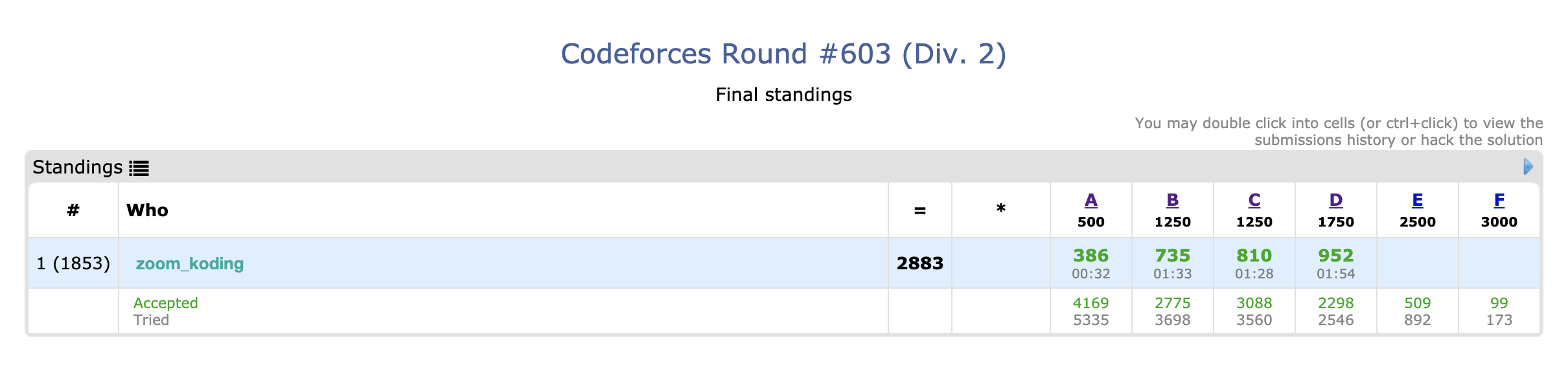## Introduction

• First time writing a Codeforces contest review in English
• I have been participating in the Codeforces rounds since this October.## A. Sweet Problem

• I thought this problem is easy to solve since its scoring is 500.
• It was tough to find a way to think of how to find the maximum day.
• My algorithm to find the max day :
• 1.Sort the input numbers.
• 2.If the smallest number, arr, is smaller than the difference between arr and arr, the answer is arr + arr.
• 3.Otherwise, some of arr is used to make arr and arr equal and then the rest of arr is evenly eaten wtih arr and arr.
• Be careful! If the rest of arr is odd, the answer should be lessened by 1.
#include <cstdio>
#include <algorithm>
using namespace std;
long long k, arr;
int main(){
scanf("%lld", &k);
while(k--){
scanf("%lld %lld %lld", &arr, &arr, &arr);
long long ans = 0;
sort(arr, arr + 3);
int dif = arr - arr;
if(dif >= arr)ans = arr + arr;
else{
if((arr - dif) % 2 == 0)ans = arr + arr - (arr - dif) / 2;
else ans = arr + arr - (arr - dif) / 2 - 1;
}
printf("%lld\n", ans);
}
}


## B. PIN Codes

• This problem can be seen hard if you don’t see the condition that n is 10.
• I just receive numbers and put them in the array.
• Array cnt is used to see the number is duplicated or not.
• To change digits of the number, I use 3 variables, x for thousands, y for hundreds and z for ones.
• If a number is duplicated, it looks for a valid number using x, y, z.
• Then, finally put the number into the answer.
#include <algorithm>
#include <cstring>
#include <cstdio>
#include <vector>
using namespace std;
int n, t, temp, num, cnt;
int main(){
scanf("%d", &t);
while(t--){
int ans = 0;
vector<int> nums;
scanf("%d", &n);
memset(cnt, 0, sizeof(cnt));
for(int i = 0; i < n; i++){
scanf("%d", &num[i]);
cnt[num[i]]++;
}
for(int i = 0; i < n; i++){
if(cnt[num[i]] == 1){
nums.push_back(num[i]);
continue;
}
ans ++;
int x = num[i] % 1000, y = num[i] - x / 100 * 100, z = num[i] - num[i] % 10;
for(int j = 0; j < 10; j++){
int temp;
temp = x + j * 1000, temp = y + j * 100, temp = z + j;
for(int k = 0; k < 3; k++){
if(!cnt[temp[k]]){
cnt[temp[k]] ++;
nums.push_back(temp[k]);
break;
}
}
}
cnt[num[i]]--;
}
printf("%d\n", ans);
for(int i = 0; i < n; i++){
if(nums[i] / 10 == 0)printf("000");
else if(nums[i] / 100 == 0)printf("00");
else if(nums[i] / 1000 == 0)printf("0");
printf("%d\n", nums[i]);
}

}
}


## C. Everyone is a Winner!

• This problem is easy if you find out a way to find numbers.
• If the number is 11, starting from 1 to the square root of 11, you will find the result of the divison.
#include <algorithm>
#include <vector>
#include <cstdio>
#include <cmath>
using namespace std;
long long n, m, t;
int main(){
scanf("%lld", &t);
while(t--){
scanf("%lld", &n);
vector<long long> ans;
long long prev = 0, p = sqrt(n);
for(long long i = 1; i <= p; i++){
ans.push_back(i);
if(i != n / i)ans.push_back(n / i);
}
sort(ans.begin(), ans.end());
printf("%lu\n0 ", ans.size() + 1);
for(long long i = 0; i < ans.size(); i++)printf("%lld ", ans[i]);
printf("\n");
}
}


• I had only 20 mins to solve this problem.
• The goal is to find the minimum number of passwords that can cover all of the list.
• This can be solved by finding letter sets that can cover more pw in the list.
• If a password is with more than one letter, union the letters in it.
• Then, count the numbers of disjoint letter sets.
#include <cstdio>
char s;
int ans, n, m, t, par, visited;
int find(int x) {
if (x == par[x])return x;
return par[x] = find(par[x]);
}
int main(){
scanf("%d", &n);
for(int i = 0; i < 26; i++)par[i] = i;
for(int i = 0; i < n; i++){
scanf("%s", s);
for(int j = 0; s[j] != '\0'; j++){
visited[s[j] - 'a'] = 1;
if(s[j + 1] == '\0')break;
int p1 = find(s[j] - 'a'), p2 = find(s[j + 1] - 'a');
if(p1 == p2)continue;
else par[p1] = p2;
}
}
for(int i = 0; i < 26; i++){
if(visited[i] == 0)continue;
if(par[i] == i)ans++;
}
printf("%d", ans);
}


If you find better ways to solve in my code or have any questions, leave a comment!